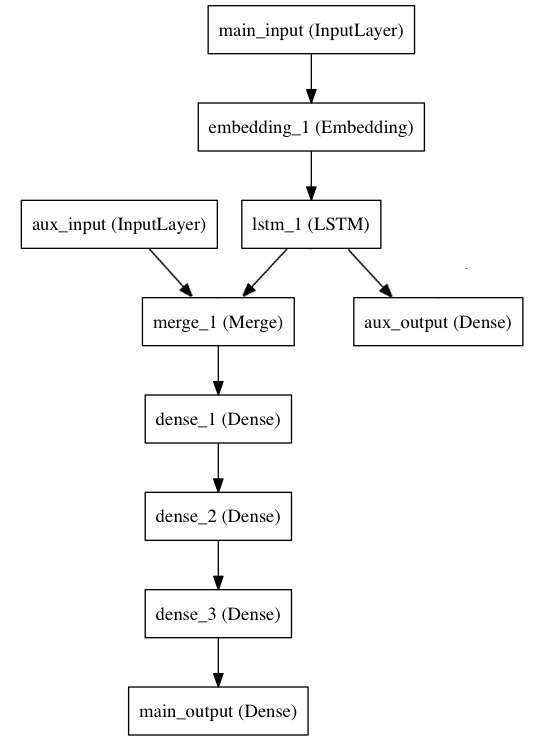# 开始使用 Keras 函数式 API

Keras 函数式 API 是定义复杂模型（如多输出模型、有向无环图或具有共享层的模型）的方法。

## 例一：全连接网络

`Sequential` 模型可能是实现这种网络的一个更好选择，但这个例子能够帮助我们进行一些简单的理解。

• 网络层的实例是可调用的，它以张量为参数，并且返回一个张量
• 输入和输出均为张量，它们都可以用来定义一个模型（`Model`
• 这样的模型同 Keras 的 `Sequential` 模型一样，都可以被训练
``````from keras.layers import Input, Dense
from keras.models import Model

# 这部分返回一个张量
inputs = Input(shape=(784,))

# 层的实例是可调用的，它以张量为参数，并且返回一个张量
output_1 = Dense(64, activation='relu')(inputs)
output_2 = Dense(64, activation='relu')(output_1)
predictions = Dense(10, activation='softmax')(output_2)

# 这部分创建了一个包含输入层和三个全连接层的模型
model = Model(inputs=inputs, outputs=predictions)
model.compile(optimizer='rmsprop',
loss='categorical_crossentropy',
metrics=['accuracy'])
model.fit(data, labels)  # 开始训练
``````

## 所有的模型都可调用，就像网络层一样

``````x = Input(shape=(784,))
# 这是可行的，并且返回上面定义的 10-way softmax。
y = model(x)
``````

``````from keras.layers import TimeDistributed

# 输入张量是 20 个时间步的序列，
# 每一个时间为一个 784 维的向量
input_sequences = Input(shape=(20, 784))

# 这部分将我们之前定义的模型应用于输入序列中的每个时间步。
# 之前定义的模型的输出是一个 10-way softmax，
# 因而下面的层的输出将是维度为 10 的 20 个向量的序列。
processed_sequences = TimeDistributed(model)(input_sequences)
``````

## 多输入多输出模型``````from keras.layers import Input, Embedding, LSTM, Dense
from keras.models import Model
import numpy as np
np.random.seed(0)  # 设置随机种子，用于复现结果

# 标题输入：接收一个含有 100 个整数的序列，每个整数在 1 到 10000 之间。
# 注意我们可以通过传递一个 "name" 参数来命名任何层。
main_input = Input(shape=(100,), dtype='int32', name='main_input')

# Embedding 层将输入序列编码为一个稠密向量的序列，
# 每个向量维度为 512。
x = Embedding(output_dim=512, input_dim=10000, input_length=100)(main_input)

# LSTM 层把向量序列转换成单个向量，
# 它包含整个序列的上下文信息
lstm_out = LSTM(32)(x)
``````

``````auxiliary_output = Dense(1, activation='sigmoid', name='aux_output')(lstm_out)
``````

``````auxiliary_input = Input(shape=(5,), name='aux_input')
x = keras.layers.concatenate([lstm_out, auxiliary_input])

# 堆叠多个全连接网络层
x = Dense(64, activation='relu')(x)
x = Dense(64, activation='relu')(x)
x = Dense(64, activation='relu')(x)

# 最后添加主要的逻辑回归层
main_output = Dense(1, activation='sigmoid', name='main_output')(x)
``````

``````model = Model(inputs=[main_input, auxiliary_input], outputs=[main_output, auxiliary_output])
``````

``````model.compile(optimizer='rmsprop', loss='binary_crossentropy',
loss_weights=[1., 0.2])
``````

``````headline_data = np.round(np.abs(np.random.rand(12, 100) * 100))
epochs=50, batch_size=32)
``````

``````model.compile(optimizer='rmsprop',
loss={'main_output': 'binary_crossentropy', 'aux_output': 'binary_crossentropy'},
loss_weights={'main_output': 1., 'aux_output': 0.2})

# 然后使用以下方式训练：
epochs=50, batch_size=32)
``````

``````model.predict({'main_input': headline_data, 'aux_input': additional_data})
``````

``````pred = model.predict([headline_data, additional_data])
``````

## 共享网络层

``````import keras
from keras.layers import Input, LSTM, Dense
from keras.models import Model

tweet_a = Input(shape=(280, 256))
tweet_b = Input(shape=(280, 256))
``````

``````# 这一层可以输入一个矩阵，并返回一个 64 维的向量
shared_lstm = LSTM(64)

# 当我们重用相同的图层实例多次，图层的权重也会被重用 (它其实就是同一层)
encoded_a = shared_lstm(tweet_a)
encoded_b = shared_lstm(tweet_b)

# 然后再连接两个向量：
merged_vector = keras.layers.concatenate([encoded_a, encoded_b], axis=-1)

# 再在上面添加一个逻辑回归层
predictions = Dense(1, activation='sigmoid')(merged_vector)

# 定义一个连接推特输入和预测的可训练的模型
model = Model(inputs=[tweet_a, tweet_b], outputs=predictions)

model.compile(optimizer='rmsprop',
loss='binary_crossentropy',
metrics=['accuracy'])
model.fit([data_a, data_b], labels, epochs=10)
``````

## 层「节点」的概念

``````a = Input(shape=(280, 256))

lstm = LSTM(32)
encoded_a = lstm(a)

assert lstm.output == encoded_a
``````

``````a = Input(shape=(280, 256))
b = Input(shape=(280, 256))

lstm = LSTM(32)
encoded_a = lstm(a)
encoded_b = lstm(b)

lstm.output
``````
``````>> AttributeError: Layer lstm_1 has multiple inbound nodes,
hence the notion of "layer output" is ill-defined.
``````

``````assert lstm.get_output_at(0) == encoded_a
assert lstm.get_output_at(1) == encoded_b
``````

`input_shape``output_shape` 这两个属性也是如此：只要该层只有一个节点，或者只要所有节点具有相同的输入/输出尺寸，那么「层输出/输入尺寸」的概念就被很好地定义，且将由 `layer.output_shape` / `layer.input_shape` 返回。但是比如说，如果将一个 `Conv2D` 层先应用于尺寸为 `(32，32，3)` 的输入，再应用于尺寸为 `(64, 64, 3)` 的输入，那么这个层就会有多个输入/输出尺寸，你将不得不通过指定它们所属节点的索引来获取它们：

``````a = Input(shape=(32, 32, 3))
b = Input(shape=(64, 64, 3))

conv = Conv2D(16, (3, 3), padding='same')
conved_a = conv(a)

# 到目前为止只有一个输入，以下可行：
assert conv.input_shape == (None, 32, 32, 3)

conved_b = conv(b)
# 现在 `.input_shape` 属性不可行，但是这样可以：
assert conv.get_input_shape_at(0) == (None, 32, 32, 3)
assert conv.get_input_shape_at(1) == (None, 64, 64, 3)
``````

## 更多的例子

### Inception 模型

``````from keras.layers import Conv2D, MaxPooling2D, Input

input_img = Input(shape=(256, 256, 3))

tower_1 = Conv2D(64, (1, 1), padding='same', activation='relu')(input_img)
tower_1 = Conv2D(64, (3, 3), padding='same', activation='relu')(tower_1)

tower_2 = Conv2D(64, (1, 1), padding='same', activation='relu')(input_img)
tower_2 = Conv2D(64, (5, 5), padding='same', activation='relu')(tower_2)

tower_3 = MaxPooling2D((3, 3), strides=(1, 1), padding='same')(input_img)
tower_3 = Conv2D(64, (1, 1), padding='same', activation='relu')(tower_3)

output = keras.layers.concatenate([tower_1, tower_2, tower_3], axis=1)
``````

### 卷积层上的残差连接

``````from keras.layers import Conv2D, Input

# 输入张量为 3 通道 256x256 图像
x = Input(shape=(256, 256, 3))
# 3 输出通道（与输入通道相同）的 3x3 卷积核
y = Conv2D(3, (3, 3), padding='same')(x)
# 返回 x + y
``````

### 共享视觉模型

``````from keras.layers import Conv2D, MaxPooling2D, Input, Dense, Flatten
from keras.models import Model

# 首先，定义视觉模型
digit_input = Input(shape=(27, 27, 1))
x = Conv2D(64, (3, 3))(digit_input)
x = Conv2D(64, (3, 3))(x)
x = MaxPooling2D((2, 2))(x)
out = Flatten()(x)

vision_model = Model(digit_input, out)

# 然后，定义区分数字的模型
digit_a = Input(shape=(27, 27, 1))
digit_b = Input(shape=(27, 27, 1))

# 视觉模型将被共享，包括权重和其他所有
out_a = vision_model(digit_a)
out_b = vision_model(digit_b)

concatenated = keras.layers.concatenate([out_a, out_b])
out = Dense(1, activation='sigmoid')(concatenated)

classification_model = Model([digit_a, digit_b], out)
``````

### 视觉问答模型

``````from keras.layers import Conv2D, MaxPooling2D, Flatten
from keras.layers import Input, LSTM, Embedding, Dense
from keras.models import Model, Sequential

# 首先，让我们用 Sequential 来定义一个视觉模型。
# 这个模型会把一张图像编码为向量。
vision_model = Sequential()

# 现在让我们用视觉模型来得到一个输出张量：
image_input = Input(shape=(224, 224, 3))
encoded_image = vision_model(image_input)

# 接下来，定义一个语言模型来将问题编码成一个向量。
# 每个问题最长 100 个词，词的索引从 1 到 9999.
question_input = Input(shape=(100,), dtype='int32')
embedded_question = Embedding(input_dim=10000, output_dim=256, input_length=100)(question_input)
encoded_question = LSTM(256)(embedded_question)

# 连接问题向量和图像向量：
merged = keras.layers.concatenate([encoded_question, encoded_image])

# 然后在上面训练一个 1000 词的逻辑回归模型：
output = Dense(1000, activation='softmax')(merged)

# 最终模型：
vqa_model = Model(inputs=[image_input, question_input], outputs=output)

# 下一步就是在真实数据上训练模型。
``````

### 视频问答模型

``````from keras.layers import TimeDistributed

video_input = Input(shape=(100, 224, 224, 3))
# 这是基于之前定义的视觉模型（权重被重用）构建的视频编码
encoded_frame_sequence = TimeDistributed(vision_model)(video_input)  # 输出为向量的序列
encoded_video = LSTM(256)(encoded_frame_sequence)  # 输出为一个向量

# 这是问题编码器的模型级表示，重复使用与之前相同的权重：
question_encoder = Model(inputs=question_input, outputs=encoded_question)

# 让我们用它来编码这个问题：
video_question_input = Input(shape=(100,), dtype='int32')
encoded_video_question = question_encoder(video_question_input)

# 这就是我们的视频问答模式：
merged = keras.layers.concatenate([encoded_video, encoded_video_question])
output = Dense(1000, activation='softmax')(merged)
video_qa_model = Model(inputs=[video_input, video_question_input], outputs=output)
``````